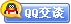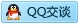##用户名 Email 自动登录 找回密码 密码 会员注册
 VIP会员，3年作业免费下 ！ 奥鹏作业，奥鹏毕业论文检测 新手作业下载教程，充值问题 没有找到答案，请在此处留言！ 2020年04月最新全国统考资料 投诉建议，加盟合作！奥鹏课程积分软件(ver:3.1)

# 北语15秋《Java语言程序设计》作业1答案辅导资料发表于 2015-11-2 09:55:56 | 显示全部楼层 |阅读模式15秋《Jv语言程序设计》作业1 一、单选题（共 10 道试题，共 100 分。） 1.  下列关于一个二维的整型数组的定义，不正确的是_______。 . int =nw int[][]; . int [][]=nw int; . int [][]=nw int; . int [][]=nw int; 谋学网： 2.  假设是int类型的变量，并初始化为1，则下列选项中，合法的一个条件语句是_______。 . if(){} . if(<<=3){} . if(=2){} . if(tru){} 谋学网： 3.  用整型数10创建一个Intgr类的对象，下列语句中，能够完成上述功能的是_______。 . Intgr i=nw Intgr(10);grLU . Intgr i=10; . int i=10; . Intgr i=Intgr (10); 谋学网： 4.  下面的程序段，方法返回的类型为_______。puli lss Rturn{ RturnTyp (yt x,oul y){ rturn (short)x/y*2; } } . oul . yt . flot . short 谋学网： 5.  下列关于基本数据类型的取值范围的描述中，正确的一项是_______。 . yt类型的范围是-128～128 . ooln类型的范围是真或假 . hr类型的范围是0～65536 . short类型的范围是-32767～32767 谋学网： 6.  下列关于变量组成部分的描述中正确的一项是_______。 . 变量名不是标识符 . 变量名是标识符 . 浮点型属于复合类型 . 变量属性分为两类，基本类型和复合类型 谋学网： 7.  下面程序段的输出结果为_______。puli lss Tst { puli stti voi min(String rgs[]){ hr 1,2,3; 1='H'; 2='\\'; 3='\115'; Systm.out.print(1); Systm.out.print(2); Systm.out.print(3); . H\M . H\\\115 . H115 . 无输出 谋学网： 8.  执行下面的程序段后，j的值为_______。puli lss Nwlss { puli stti voi min(String rgs[]){ int i=0x00; int j=~i; Systm.out.print(j); } } . 14 . -14 . -15 . 15 谋学网： 9.  下面的程序段运行结果为_______。puli lss xmplStringuffr { puli stti voi min(String rgs[]){ Stringuffr s=nw Stringuffr("tst"); Systm.out.println("lngth="+s.lngth()); Systm.out.println("hrt="+s.hrt(3)); . lngth=20 hrt=t . lngth=20 hrt=s . lngth=4 hrt=s . lngth=4 hrt=t 谋学网： 10.  下面程序段的输出结果为puli lss Tst { puli stti voi min(String rgs[]){ int []={1,2,3},[]=nw int ; Systm.rryopy(, 0, , 0, 3); Systm.out.println("="++" ="+); =10; Systm.out.println(" . =1 =1 =10 =1 . =10 =1 =10 =1 . =1 =10 =1 =10 . =10 =10 =1 =1 谋学网：

### 本帖子中包含更多资源

x发表于 2015-12-2 09:38:12 | 显示全部楼层回复 支持 反对发表于 2015-12-2 09:39:25 | 显示全部楼层回复 支持 反对发表于 2015-12-2 09:53:42 | 显示全部楼层回复 支持 反对
 本版积分规则 回帖并转播 回帖后跳转到最后一页客服一客服二客服三客服四微信客服扫一扫